# Part 5: Normal Distribution | Free Worksheet and Solutions

In this article, we go through the definition of normal distribution, their key parameters, the z-score and empirical rule and provide examples, concept-check questions and solutions.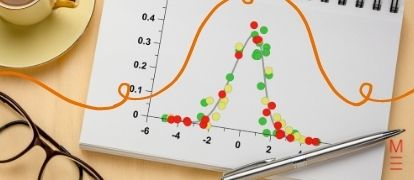So you know how to solve continuous random variables and functions, but you’re still unsure about normal distribution. Don’t worry! This article will guide you through everything you need to know about normal distribution.

## Year 12 Advanced Mathematics: Normal distribution

When scientists began taking rigorous statistical measurements of natural phenomena, a number of common patterns emerged. Of these, the most common distribution had sample values deviating from a mean value in predictable proportions.

This makes the so called “normal distribution” a useful tool to study and compare data sets with.

This blog article will explain the Normal Distribution and several properties of the normal distribution that can be applied to various data sets.

This resource will help by supplementing the new Year 12 Syllabus to provide an introduction or a refresher on the Normal distribution.

## NESA Syllabus outcomes

S3.2: The normal distribution

Students:

• Identify the numerical and graphical properties of data that is normally distributed.
• Calculate probabilities and quantiles with a given normal distribution using technology and otherwise, and use these to solve practical problems
• Identify contexts that are suitable for modelling by normal random variables, e.g. the height of a group of students.
• Recognise features of the graph of the probability density function of the normal distribution with mean  and standard deviation , and the use of the standard normal distribution
• Visually represent probabilities by shading areas under the normal curve, e.g. identifying the value above which the top 10% of data lies
• Understand and calculate the z-score (standardised score) corresponding to a particular value in a dataset
• Use the formula $$z = \frac{x – \mu}{\sigma}$$, where $$\mu$$ is the mean and $$\sigma$$ is the standard deviation
• Describe the $$z – score$$ as well as the number of standard deviations a value lies above or below the mean
• Use z-scores to compare scores from different datasets, for example comparing students’ subject examination scores
• Use collected data to illustrate the empirical rules for normally distributed random variables
• Apply the empirical rule to a variety of problems
• Sketch the graphs of  $$f(x) = e^{-x^2}$$ and the probability density function for the normal distribution $$f(x) = \frac{1}{\sigma \sqrt{2\pi}} e^{-\frac{(x-\mu)^2}{2\sigma^2}}$$ using technology
• Verify, using the trapezoidal rule, the results concerning the areas under the normal curve
• Use z-scores to identify probabilities of events less or more extreme than a given event
• Use statistical tables to determine probabilities
• Use technology to determine probabilities
•  Use z-scores to make judgements related to outcomes of a given event or sets of data.

## Assumed knowledge

To understand the normal distribution, you should have a good understanding of:

• What a continuous random variable is;
• What a probability density function is;
• Statistical terms, including
• The mean;
• The standard deviation;
• Exponential functions
• Calculus, including the trapezoidal rule.

Need a refresher on continuous random variables? Check out our post on Continuous Random Variables.

## The Normal Distribution: Definition and examples

The Normal Distribution is a common distribution of a continuous random variable.

A quick refresher: A distribution of a continuous random variable describes the probability that a given variable (whether measured or chosen out of an existing dataset) will fall in a certain range.

A normal distribution is distinguished from other probability distributions by its particular shape: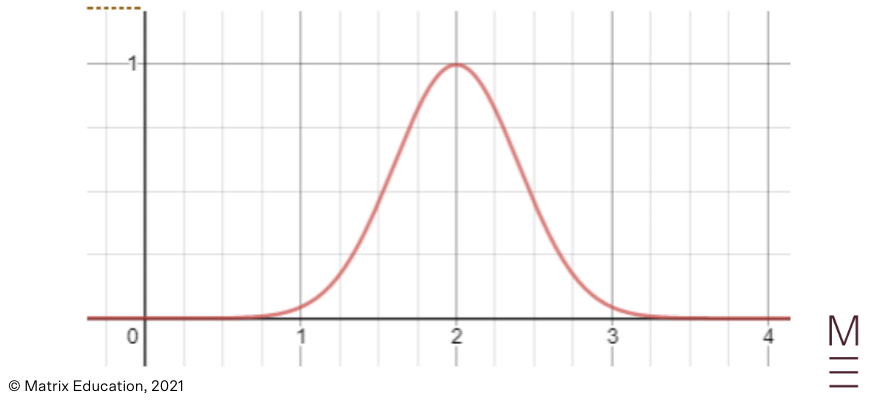Normal distribution

A binomial distribution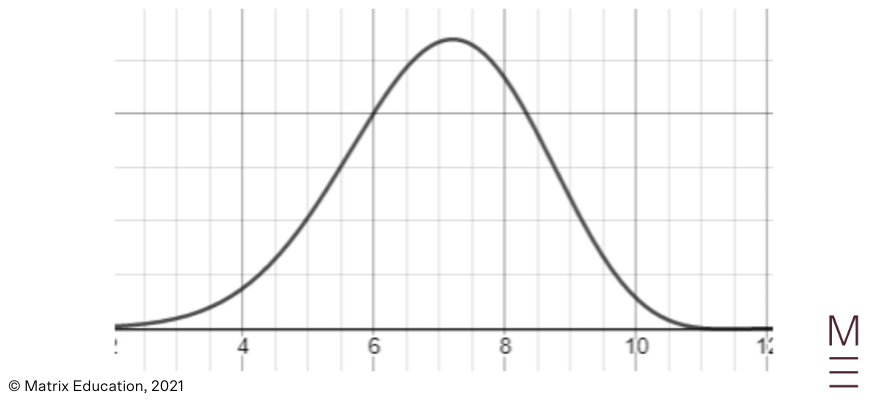Binomial distribution

A uniform distribution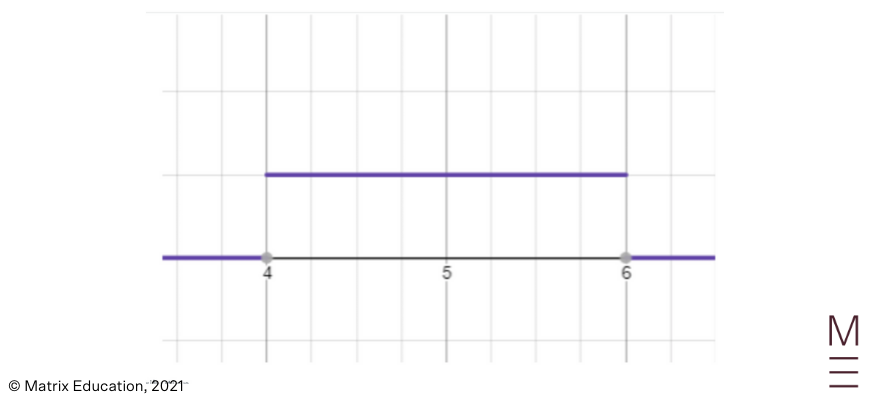Uniform distribution

Normal distributions are typically suitable for measurements of a physical quantity e.g. height or weight in a population where there is some variation.

Typical examples of normal distributions might be the height of a class of students, or the weight of a population of marmots.

The normal approximation may also be applied to financial models, for example in the stock market.

When describing the shape of the Normal Distribution, we note that the shape is symmetrical about the mean.

## Key parameters of the Normal Distribution

Although all normal distributions have the same shape, they can be shifted or stretched to fit different sets of measurements depending on two key parameters.

### Mean

The mean of the measurements determines where the peak (also the centre) of the normal distribution lies.

The mean is usually denoted using the symbol $$\mu$$.

For example, in the graphs below, the first curve has a mean of 2 and the second has a mean of 4.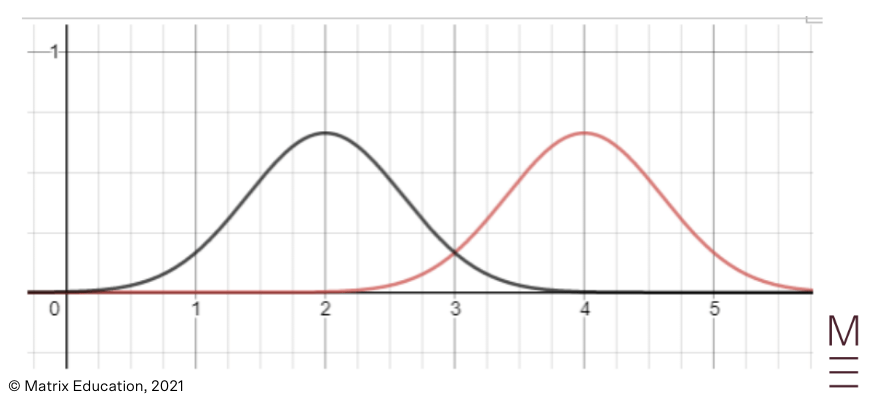### Standard deviation

The standard deviation of the measurements determines how flat the normal distribution is.

The standard deviation is typically denoted using the symbol $$\sigma$$..

A high standard deviation results in a flatter curve, compared to a lower standard deviation.

For example, in the graphs below, the red curve has a standard deviation of 0.5 and the black curve has a standard deviation of 1.

Both are still normally distributed however!## The Normal Distribution Function

A function can be derived for the normal distribution using calculus.

Formally, a normal distribution is one in which the likelihood of a value occurring decreases proportional to the distance between the mean and the value, and also decreases proportional to the likelihood itself.

This results in a differential equation which can be solved to produce the following probability density function (the derivation of which is typically covered in university):

• $$f(x) = \frac{1}{\sigma \sqrt{2\pi}} e^{-\frac{(x-\mu)^2}{2\sigma^2}}$$
• Note that $$\sigma$$ and $$\mu$$ are the standard deviation and mean respectively.

If you use Desmos or another graphing calculator to plot the function above (specifying $$\sigma$$ and $$\mu$$, you will get the normal distribution curve as seen in the screenshots above.

Try our free worksheet!## The z-score and empirical rule

If we have a normally distributed set, how can we quickly compare how far a point is from the centre?

Say we have a point that is 10 from the centre. This would be a lot if the standard deviation was 1; but would be relatively small if the standard deviation was 100.

For this reason, we should adjust the distance from the centre by the standard deviation by dividing by the standard deviation.

This results in the z-score, which is given by the formula:

$$z – score = \frac{x-\mu}{\sigma}$$

Where $$x$$ is a particular data point, $$\sigma$$ is the standard deviation, and $$\mu$$ is the mean.

Note that this means if the value is bigger than the mean, then the z-score is positive; but for values less than the mean the z-score will be negative.

Since the standard deviation has the same shape but is flattened by the standard deviation, when we divide by the standard deviation, this reverses the flattening effect for the single data point.

Therefore, we can use the z-score to make inferences about the particular data point based on the general shape of the normal distribution.

A given z-score corresponds to a given rarity; and for z scores of 1, 2 and 3, we have:

 z-score Percentage of values that are less than that z-score +1 68% +2 95% +3 99.7%

This set of z-scores should be remembered, and collectively they are known as the Empirical rule.

Be prepared to interpret similar tables as well in an exam!

## Concept Check Questions

After a haircut, a curious student collects their hair and finds that the lengths of the hair follow the normal distribution. The mean hair length is 20cm, and the standard deviation is 5cm.

1. Sketch the distribution of hairs on a graph.
2. The student makes some statements. Which of these are true?
1. Most of the hairs are exactly 20cm.
2. The interquartile range of the hair is less than 20cm.
3. No hair is more than 50cm long.
3. What is the z-score of a hair that is 18cm long?
4. What proportion of hairs are less than 10cm long?
5. Complete the statement: There are just as many hairs that are more than 22cm, as there are hairs that are less than $$x$$ cm.

## Concept Check Solutions

1.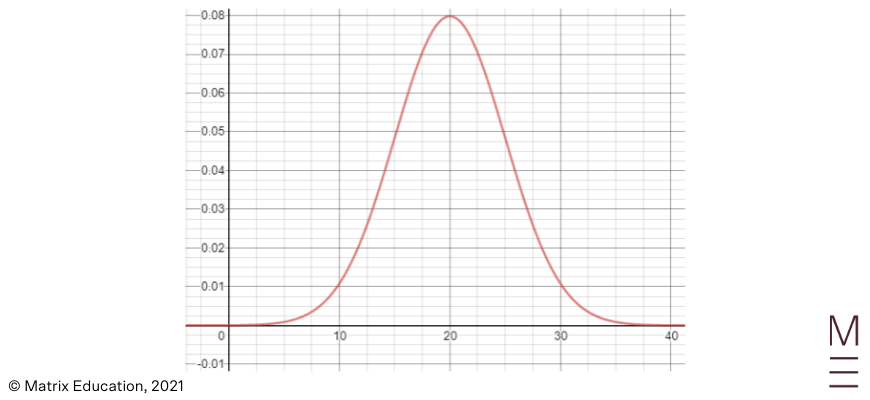2.

a. False: Even though the mean is 20, there do not have to be any hairs that are exactly 20cm long.

b. True: The interquartile range is the range between 25% and 75% probability; and we know that 75% is between 1 z-score and 2 z-score, i.e. between 25 and 30. Similarly, 25% is between -1 z-score and -2 z score, i.e. between 15 and 10. Therefore, the interquartile range is at most 30-10=20cm.

c. False: although it is highly likely that no hair is longer than even 40cm, there is a possibility that there is a hair that is 50cm long.

3. The z-score is $$\frac{18-20}{5} = \frac{-2}{5} = -0.4$$

4. 10 corresponds to a z-score of -2. We know that 95% of hairs are shorter than 2 z-scores more than 20cm; and because of symmetry, 95% of hairs are longer than -2 z-score. This leaves 5% of hairs less than 10cm.

5. Again, because of symmetry, we have just as many hairs that are more than $$z = \frac{22-2}{5} = 0.4$$ away from the mean, as there are hairs that are less than $$x = \mu – 0.4 \sigma = 20 – 2 = 18$$ cm.

## Are you struggling to grasp Normal Distribution?© Matrix Education and www.matrix.edu.au, 2021. Unauthorised use and/or duplication of this material without express and written permission from this site’s author and/or owner is strictly prohibited. Excerpts and links may be used, provided that full and clear credit is given to Matrix Education and www.matrix.edu.au with appropriate and specific direction to the original content.